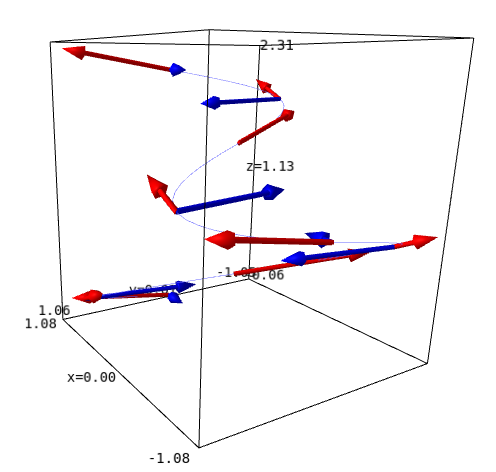# Plot velocity and acceleration vectors of a space curve

I'm having trouble plotting the velocity and acceleration vectors of the following space curve (r3).

May anyone help guide me in the right direction to solve this problem?

var('t')
r3(t) = (cos(t/2), sin(t), t/6)
v3 = diff(r3(t),t)
a3 = diff(v3,t)
curve3 = parametric_plot3d(r3(t),(t,0,4*pi),thickness=0.5)
velvecs = Graphics()
accvecs = Graphics()
for k in range(9):
velvecs += arrow(r3(k*pi/2),r3(k*pi/2)+v3(k*pi/2),color='red') #Line 26
accvecs += arrow(r3(k*pi/2),r3(k*pi/2)+a3(k*pi/2),color='blue')
show(velvecs+accvecs+curve3,axes=False)

edit retag close merge delete

Sort by » oldest newest most votedThe problem is that calling v3(k*pi/2) will try to call each component of v3 with that positional argument, but the last component of v3 is 1/6, so calling it with a positional argument is not supported. You can call it with a keyword argument instead, by using v3(t=k*pi/2). To be even more explicit, you can write v3.subs(t=k*pi/2). Similarly for a3.

Or, what you probably meant to do: make v3 callable too:

v3 = diff(r3,t)
a3 = diff(v3,t)


With both approaches, your code works:more